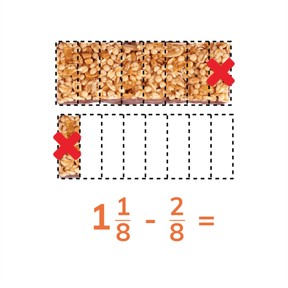Subtracting mixed numbers whose fractions have common denominators

# Subtracting mixed numbers whose fractions have common denominators8,000 schools use Gynzy92,000 teachers use Gynzy1,600,000 students use Gynzy

## General

Students learn to subtract mixed numbers whose fractions have common denominators.

## Common core standard(s)

CCSS.Math.Content.4.NF.A

## Relevance

Discuss with students that it is useful to subtract mixed numbers whose fractions have common denominators so you can determine how much you have left.

## Introduction

The interactive whiteboard shows a selection of fractions greater than 1 and mixed numbers. Ask students to convert the fractions greater than 1 and them match fractions by circling them in the same color.

## Development

Tell students that fractions with a common denominator have the same denominator. When fractions have a common denominator, you can add the numerators together. Show the example on the interactive whiteboard and explain to students that the subtraction problem takes away a unit from a whole number. There is 1 1//3 of a cake. Tell students that you can convert this into a fraction greater than 1, and it becomes 4//3. Now 2//3 of the cake is eaten. You can subtract the numerators to determine how much is left, in this case 2//3. Show students more subtraction problems with visual support of a fraction bar. Encourage them to convert the mixed number into a fraction greater than 1 to simplify their subtraction. Fractions that don't have visual support can be subtracted in the same way by first converting the mixed number into a fraction greater than 1 and then subtracting numerators. Remind students to be sure to check at the end of their subtraction to see if they have a fraction greater than 1 that they can convert into a mixed number. Have students practice the following problems individually or in pairs. Finally explain how to solve fraction story problems. They must find the fractions in the word problems and determine the equation before solving the problem. Practice as a class and then have students work individually or in pairs.

To check that students are able to subtract mixed numbers whose fractions have common denominators by asking the following question:
- How can you subtract a mixed number?

## Guided practice

Students are given subtraction problems with visual support, then without visual support, and finally are given fraction word problems to solve.

## Closing

Discuss with students that it is important to be able to subtract mixed numbers whose fractions have a common denominator so that they are able to determine how much they have left. Have students match the mixed number subtraction problems to the answers shown on the interactive whiteboard. One answer will be left over. Have students come up with a subtraction problem that has that number as a difference.

## Teaching tips

Students who have difficulty subtracting mixed numbers whose fractions have a common denominator can first practice subtracting fractions with a common denominator. Once they feel more comfortable, they can work on converting fractions greater than 1 to mixed numbers and vice versa. That should make them comfortable subtracting the mixed numbers.

### The online teaching platform for interactive whiteboards and displays in schools

• Save time building lessons

• Manage the classroom more efficiently

• Increase student engagement